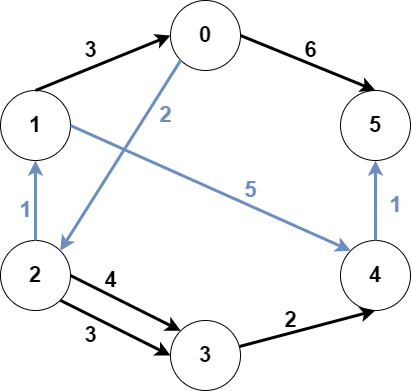2203. Minimum Weighted Subgraph With the Required Paths
Hard
574
15

You are given an integer `n` denoting the number of nodes of a weighted directed graph. The nodes are numbered from `0` to `n - 1`.

You are also given a 2D integer array `edges` where `edges[i] = [fromi, toi, weighti]` denotes that there exists a directed edge from `fromi` to `toi` with weight `weighti`.

Lastly, you are given three distinct integers `src1`, `src2`, and `dest` denoting three distinct nodes of the graph.

Return the minimum weight of a subgraph of the graph such that it is possible to reach `dest` from both `src1` and `src2` via a set of edges of this subgraph. In case such a subgraph does not exist, return `-1`.

A subgraph is a graph whose vertices and edges are subsets of the original graph. The weight of a subgraph is the sum of weights of its constituent edges.

Example 1:```Input: n = 6, edges = [[0,2,2],[0,5,6],[1,0,3],[1,4,5],[2,1,1],[2,3,3],[2,3,4],[3,4,2],[4,5,1]], src1 = 0, src2 = 1, dest = 5
Output: 9
Explanation:
The above figure represents the input graph.
The blue edges represent one of the subgraphs that yield the optimal answer.
Note that the subgraph [[1,0,3],[0,5,6]] also yields the optimal answer. It is not possible to get a subgraph with less weight satisfying all the constraints.
```

Example 2:```Input: n = 3, edges = [[0,1,1],[2,1,1]], src1 = 0, src2 = 1, dest = 2
Output: -1
Explanation:
The above figure represents the input graph.
It can be seen that there does not exist any path from node 1 to node 2, hence there are no subgraphs satisfying all the constraints.
```

Constraints:

• `3 <= n <= 105`
• `0 <= edges.length <= 105`
• `edges[i].length == 3`
• `0 <= fromi, toi, src1, src2, dest <= n - 1`
• `fromi != toi`
• `src1`, `src2`, and `dest` are pairwise distinct.
• `1 <= weight[i] <= 105`
Accepted
9.4K
Submissions
25.9K
Acceptance Rate
36.1%

Seen this question in a real interview before?
1/4
Yes
No

Discussion (0)

Related Topics